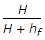# Mechanical Engineering - Hydraulics and Fluid Mechanics

### Exercise :: Hydraulics and Fluid Mechanics - Section 2

1.

A compound pipe of diameter d1, d2 and d3 having lengths l1, l2 and l3 is to be replaced by an equivalent pipe of uniform diameter d and of the same length (l) as that of the compound pipe. The size of the equivalent pipe is given by

 A.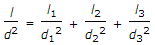B.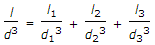C.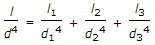D.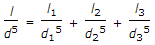Explanation:

No answer description available for this question. Let us discuss.

2.

The velocity corresponding to Reynold number of 2800, is called

 A. sub-sonic velocity B. super-sonic velocity C. lower critical velocity D. higher critical velocity

Explanation:

No answer description available for this question. Let us discuss.

3.

When the pipes are in series, the total head loss is equal to the sum of the head loss in each pipe.

 A. Yes B. No

Explanation:

No answer description available for this question. Let us discuss.

4.

Whenever a plate is held immersed at some angle with the direction of flow of the liquid, it is subjected to some pressure. The component of this pressure, at right angles to the direction of flow of the liquid is known as lift.

 A. True B. False

Explanation:

No answer description available for this question. Let us discuss.

5.

The efficiency of power transmission through pipe is (where H = Total supply head, and hf = Head lost due to friction in the pipe)

 A.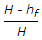B.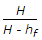C.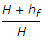D.